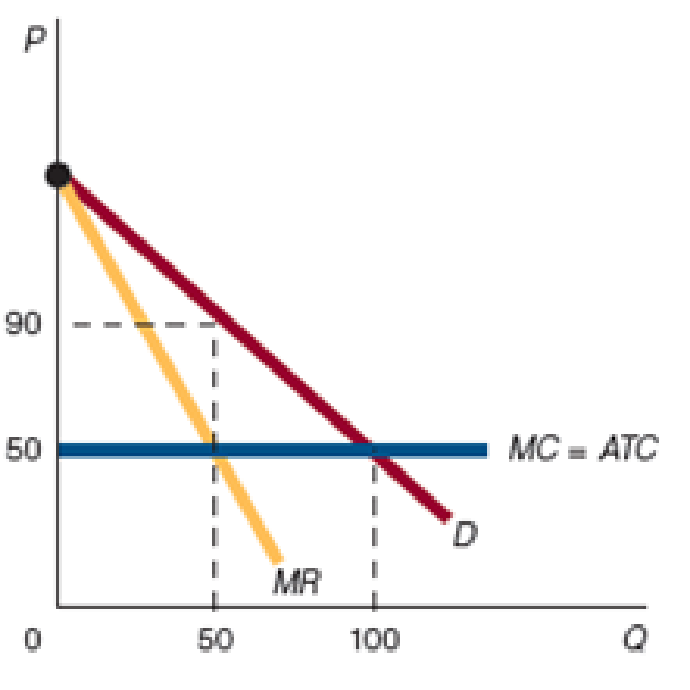Chapter 10, Problem 4WNG

Chapter
Section
Textbook Problem

If the market is perfectly competitive, how much does profit equal?To determine

Profit of a perfectly competitive market.

Explanation

The demand curve of a perfectly competitive market is horizontal, where price, average revenue marginal cost and average cost are all equal...

Still sussing out bartleby?

Check out a sample textbook solution.

See a sample solution

The Solution to Your Study Problems

Bartleby provides explanations to thousands of textbook problems written by our experts, many with advanced degrees!

Get Started

Find more solutions based on key concepts